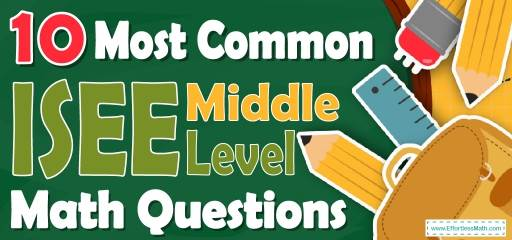# 10 Most Common ISEE Middle-Level Math QuestionsPreparing for the ISEE Middle-Level Math test? Want a preview of the most common mathematics questions on the ISEE Middle-Level Math test? If so, then you are in the right place.

The mathematics section of ISEE Middle Level can be a challenging area for many test-takers, but with enough patience, it can be easy and even enjoyable!

Preparing for the ISEE Middle-Level Math test can be a nerve-wracking experience. Learning more about what you’re going to see when you take the ISEE Middle Level can help to reduce those pre-test jitters. Here’s your chance to review the 10 most common ISEE Middle-Level Math questions to help you know what to expect and what to practice most. Try these 10 most common ISEE Middle-Level Math questions to hone your mathematical skills and to see if your math skills are up to date on what’s being asked on the exam or if you still need more practice.

Make sure to follow some of the related links at the bottom of this post to get a better idea of what kind of mathematics questions you need to practice.

## 10 Sample ISEE Middle-Level Math Practice Questions

1- The perimeter of the trapezoid below is 54. What is its area?

☐A. $$252 \space cm^2$$

☐B. $$234 \space cm^2$$

☐C. $$216 \space cm^2$$

☐D. $$130 \space cm^2$$

2- The price of a laptop is decreased by $$10\%$$ to $360. What is its original price? ☐A.$320

☐B. $380 ☐C.$390

☐D. $400 3- Find $$\frac{1}{4}$$ of $$\frac{2}{5}$$ of 120? ☐A. 16 ☐B. 12 ☐C. 8 ☐D. 4 4- Solve. $$\frac{(-48 × 0.5)}{6}$$? ☐A. 16 ☐B. $$-4$$ ☐C. $$-16$$ ☐D. 4 5- The score of Emma was half as that of Ava and the score of Mia was twice that of Ava. If the score of Mia was 60, what is the score, Emma? ☐A. 15 ☐B. 18 ☐C. 20 ☐D. 30 6- The area of a circle is $$64 π$$. What is the circumference of the circle? ☐A. $$8π$$ ☐B. $$16π$$ ☐C. $$32π$$ ☐D. $$64π$$ 7- What is the value of $$x$$ in the following figure? ☐A. $$25^\circ$$ ☐B. $$65^\circ$$ ☐C. $$115^\circ$$ ☐D. $$155^\circ$$ 8- Two-thirds of 18 is equal to $$\frac{2}{5}$$ of what number? ☐A. 60 ☐B. 20 ☐C. 12 ☐D. 30 9- In five successive hours, a car travels 40 km, 45 km, 50 km, 35 km, and 55 km. In the next five hours, it travels with an average speed of 50 km per hour. Find the total distance the car traveled in 10 hours. ☐A. 425 km ☐B. 450 km ☐C. 475 km ☐D. 500 km 10- Mary buys 8 pens for$6. What is the unit rate?

☐A. $0.75 pen ☐B.$0.70 pen

☐C. $0.65 pen ☐D.$0.50 pen

## Best ISEE Middle Level  Math Prep Resource for 2022

Therefore, the missing side (height) is $$= 54 – 18 – 12 – 14 = 10$$
A$$= \frac{1}{2}h (b_1 + b_2) = \frac{1}{2} (10) (12 + 14) = 130$$
Let $$x$$ be the original price. If the price of a laptop is decreased by $$10\%$$ to $360, then: $$90 \% \space of \space x=360⇒ 0.90x=360 ⇒ x=360÷0.90=400$$ 3- B $$\frac{2}{5}$$ of $$120=\frac{2}{5}×120=48$$ $$\frac{1}{4}$$ of $$48=\frac{1}{4}×48=12$$ 4- B $$\frac{-48×0.5}{6}=-\frac{48×\frac{1}{2}}{6}=-\frac{\frac{48}{2}}{6}=-\frac{48}{12}=-4$$ 5- A If the score of Mia was 60, therefore the score of Ava is 30. Since the score of Emma was half as that of Ava, therefore, the score of Emma is 15. 6- B Use the formula of areas of circles. Area of a circle $$=πr^2 ⇒ 64 π = πr^2 ⇒ 64 = r^2 ⇒ r = 8$$ Radius of the circle is 8. Now, use the circumference formula: Circumference $$= 2πr = 2π (8) = 16π$$ 7- D $$x=180^\circ-25^\circ=155^\circ$$ 8- D Let $$x$$ be the number. Write the equation and solve for $$x$$. $$\frac{2}{3}×18= \frac{2}{5}$$ $$x ⇒\frac{2×18}{3}=\frac{2x}{5}$$, use cross multiplication to solve for ?. $$5×36=2x×3 ⇒180=6x ⇒ x=30$$ 9- C Add the first 5 numbers. $$40 + 45 + 50 + 35 + 55 = 225$$ To find the distance traveled in the next 5 hours, multiply the average by number of hours. Distance = Average $$×$$ Rate $$= 50 × 5 = 250$$ Add both numbers. $$250 + 225 = 475$$ 10- A Unit rate $$=\frac{6}{8}=0.75$$ Looking for the best resource to help you succeed on the ISEE Middle-Level Math test? ## The Best Books to Ace the ISEE Middle Level Math Test ## Related to This Article ### More math articles ### What people say about "10 Most Common ISEE Middle-Level Math Questions - Effortless Math: We Help Students Learn to LOVE Mathematics"? No one replied yet. X 23% OFF Limited time only! Save Over 23% SAVE$5
It was $21.99 now it is$16.99